The MEANS Procedure

# Statistical Computations: MEANS Procedure

PROC MEANS uses single-pass algorithms to compute the moment statistics (such as mean, variance, skewness, and kurtosis). See Keywords and Formulas for the statistical formulas.

The computational details for confidence limits, hypothesis test statistics, and quantile statistics follow.

With the keywords CLM, LCLM, and UCLM, you can compute confidence limits for the mean. A confidence limit is a range, constructed around the value of a sample statistic, that contains the corresponding true population value with given probability (ALPHA=) in repeated sampling.

A two-sided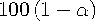% confidence interval for the mean has upper and lower limits
where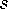is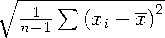and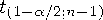is the (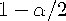) critical value of the Student's t statistics with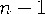degrees of freedom.

A one-sided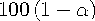% confidence interval is computed as
A two-sided% confidence interval for the standard deviation has lower and upper limits
where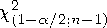and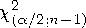are the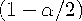and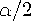critical values of the chi-square statistic with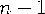degrees of freedom. A one-sided% confidence interval is computed by replacingwith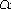.

A% confidence interval for the variance has upper and lower limits that are equal to the squares of the corresponding upper and lower limits for the standard deviation.

If you use the WEIGHT statement or WEIGHT= in a VAR statement and the default value of VARDEF=, which is DF, the% confidence interval for the weighted mean has upper and lower limits
where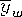is the weighted mean,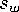is the weighted standard deviation,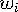is the weight for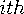observation, and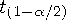is thecritical value for the Student's t distribution withdegrees of freedom.

PROC MEANS calculates the t statistic as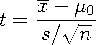where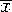is the sample mean,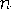is the number of nonmissing values for a variable, andis the sample standard deviation. Under the null hypothesis, the population mean equals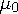. When the data values are approximately normally distributed, the probability under the null hypothesis of a t statistic as extreme as, or more extreme than, the observed value (the p-value) is obtained from the t distribution withdegrees of freedom. For large, the t statistic is asymptotically equivalent to a z test.

When you use the WEIGHT statement or WEIGHT= in a VAR statement and the default value of VARDEF=, which is DF, the Student's t statistic is calculated as
where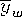is the weighted mean,is the weighted standard deviation, and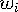is the weight for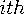observation. The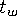statistic is treated as having a Student's t distribution withdegrees of freedom. If you specify the EXCLNPWGT option in the PROC statement, then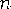is the number of nonmissing observations when the value of the WEIGHT variable is positive. By default,is the number of nonmissing observations for the WEIGHT variable.

The options QMETHOD=, QNTLDEF=, and QMARKERS= determine how PROC MEANS calculates quantiles. QNTLDEF= deals with the mathematical definition of a quantile. See Quantile and Related Statistics. QMETHOD= deals with the mechanics of how PROC MEANS handles the input data. The two methods are

OS

reads all data into memory and sorts it by unique value.

P2

accumulates all data into a fixed sample size that is used to approximate the quantile.

If data set A has 100 unique values for a numeric variable X and data set B has 1000 unique values for numeric variable X, then QMETHOD=OS for data set B will take 10 times as much memory as it does for data set A. If QMETHOD=P2, then both data sets A and B will require the same memory space to generate quantiles.

The QMETHOD=P2 technique is based on the piecewise-parabolic (P²) algorithm invented by Jain and Chlamtac (1985). P² is a one-pass algorithm to determine quantiles for a large data set. It requires a fixed amount of memory for each variable for each level within the type. However, using simulation studies, reliable estimations of some quantiles (P1, P5, P95, P99) cannot be possible for some data sets such as data sets with heavily tailed or skewed distributions.

If the number of observations is less than the QMARKERS= value, then QMETHOD=P2 produces the same results as QMETHOD=OS when QNTLDEF=5. To compute weighted quantiles, you must use QMETHOD=OS.Previous Page | Next Page | Top of Page